# How to Calculate and Solve for Temperature of Solidifying Metals | Solidification of Metals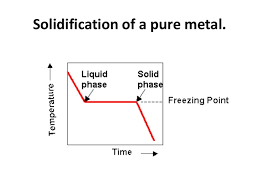The image above represents temperature of solidifying metals.

To compute for temperature of solidifying metals, three essential parameters are needed and these parameters are Surface Temperature (T), Melting Temperature of Metal (Tm) and Initial Temperature of Environment or Mould (To).

The formula for calculating temperature of solidifying metals:

Tsm = T – Tm / To – Tm

Where:

Tsm = Temperature of Solidifying Metals
T = Surface Temperature
Tm = Melting Temperature of Metal
To = Initial Temperature of Environment or Mould

Let’s solve an example;
Find the temperature of solidifying metals when the surface temperature is 12, the melting temperature of metal is 9 and the initial temperature of environment or mould is 10.

This implies that;

T = Surface Temperature = 12
Tm = Melting Temperature of Metal = 9
To = Initial Temperature of Environment or Mould = 10

Tsm = T – Tm / To – Tm
Tsm = 12 – 9 / 10 – 9
Tsm = 3 / 1
Tsm = 3

Therefore, the temperature of solidifying metals is 3 K.

Calculating for Surface Temperature when the Temperature of Solidifying Metals, the Melting Temperature of Metal and the Initial Temperature of Environment or Mould is Given.

T = Tsm (To – Tm) + Tm

Where;

T = Surface Temperature
Tsm = Temperature of Solidifying Metals
Tm = Melting Temperature of Metal
To = Initial Temperature of Environment or Mould

Let’s solve an example;
Find the surface temperature when the temperature of solidifying metals is 10, the melting temperature of metal is 8 and the initial temperature of environment or mould is 14.

This implies that;

Tsm = Temperature of Solidifying Metals = 10
Tm = Melting Temperature of Metal = 8
To = Initial Temperature of Environment or Mould = 14

T = Tsm (To – Tm) + Tm
T = 10 (14 – 8) + 8
T = 10 (6) + 8
T = 68

Therefore, the surface temperature is 68 K.

Calculating for Initial Temperature of Environment or Mould when the Temperature of Solidifying Metals, the Surface Temperature and the Melting Temperature of Metal is Given.

To = (T – Tm / Tsm) + Tm

Where;

To = Initial Temperature of Environment or Mould
Tsm = Temperature of Solidifying Metals
T = Surface Temperature
Tm = Melting Temperature of Metal

Let’s solve an example;
Find the initial temperature of environment or mould when the temperature of solidifying metals is 20, the surface temperature is 30 and the melting temperature of metal is 10.

This implies that;

Tsm = Temperature of Solidifying Metals = 20
T = Surface Temperature = 30
Tm = Melting Temperature of Metal = 10

To = (T – Tm / Tsm) + Tm
To = (30 – 10 / 20) + 10
To = (20 / 20) + 10
To = 1 + 10
To = 11

Therefore, the initial temperature of environment or mould is 11 K.

Nickzom Calculator – The Calculator Encyclopedia is capable of calculating the temperature of solidifying metals.

To get the answer and workings of the temperature of solidifying metals using the Nickzom Calculator – The Calculator Encyclopedia. First, you need to obtain the app.

You can get this app via any of these means:

To get access to the professional version via web, you need to register and subscribe for NGN 2,000 per annum to have utter access to all functionalities.
You can also try the demo version via https://www.nickzom.org/calculator

Apple (Paid) – https://itunes.apple.com/us/app/nickzom-calculator/id1331162702?mt=8
Once, you have obtained the calculator encyclopedia app, proceed to the Calculator Map, then click on Materials and Metallurgical under Engineering.Now, Click on Solidification of Metal under Materials and Metallurgical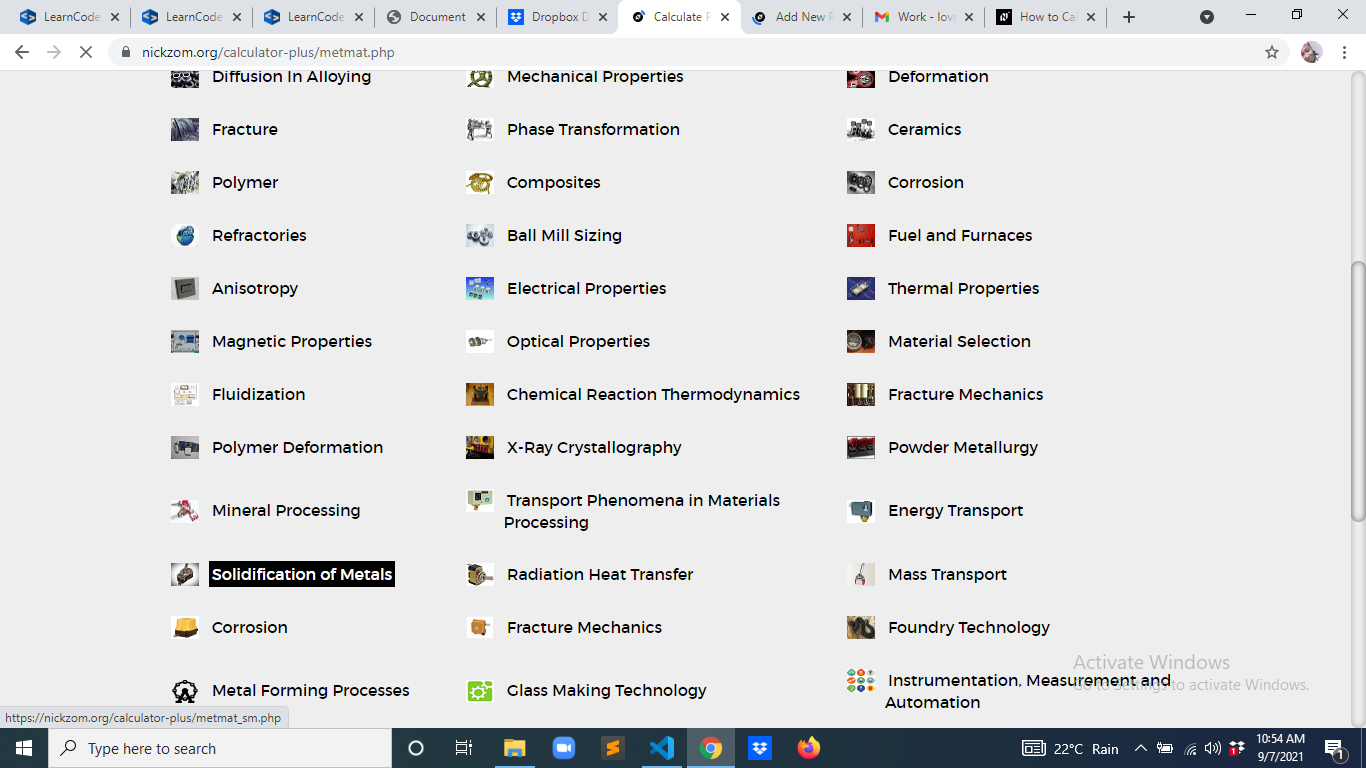Now, Click on Temperature of Solidifying Metals under Solidification of Metal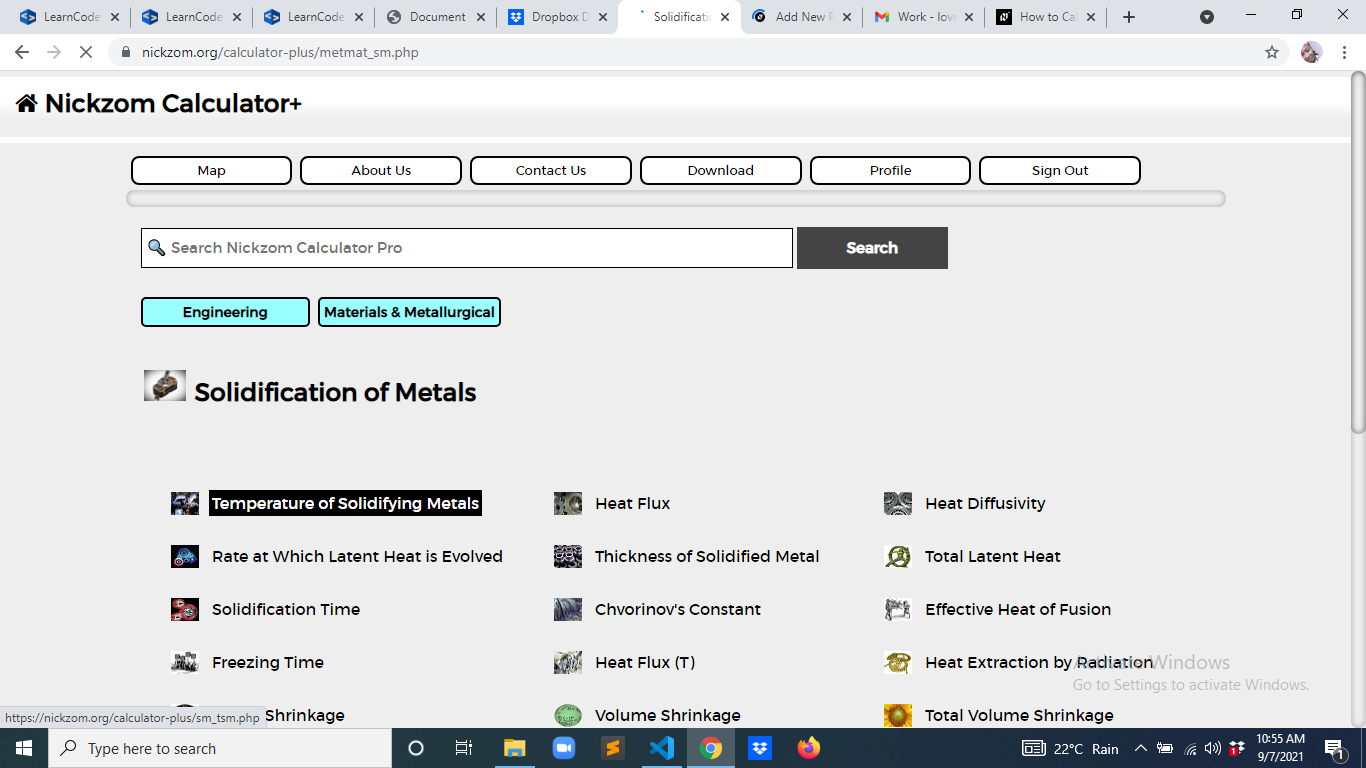The screenshot below displays the page or activity to enter your values, to get the answer for the temperature of solidifying metals according to the respective parameter which is the Surface Temperature (T), Melting Temperature of Metal (Tm) and Initial Temperature of Environment or Mould (To).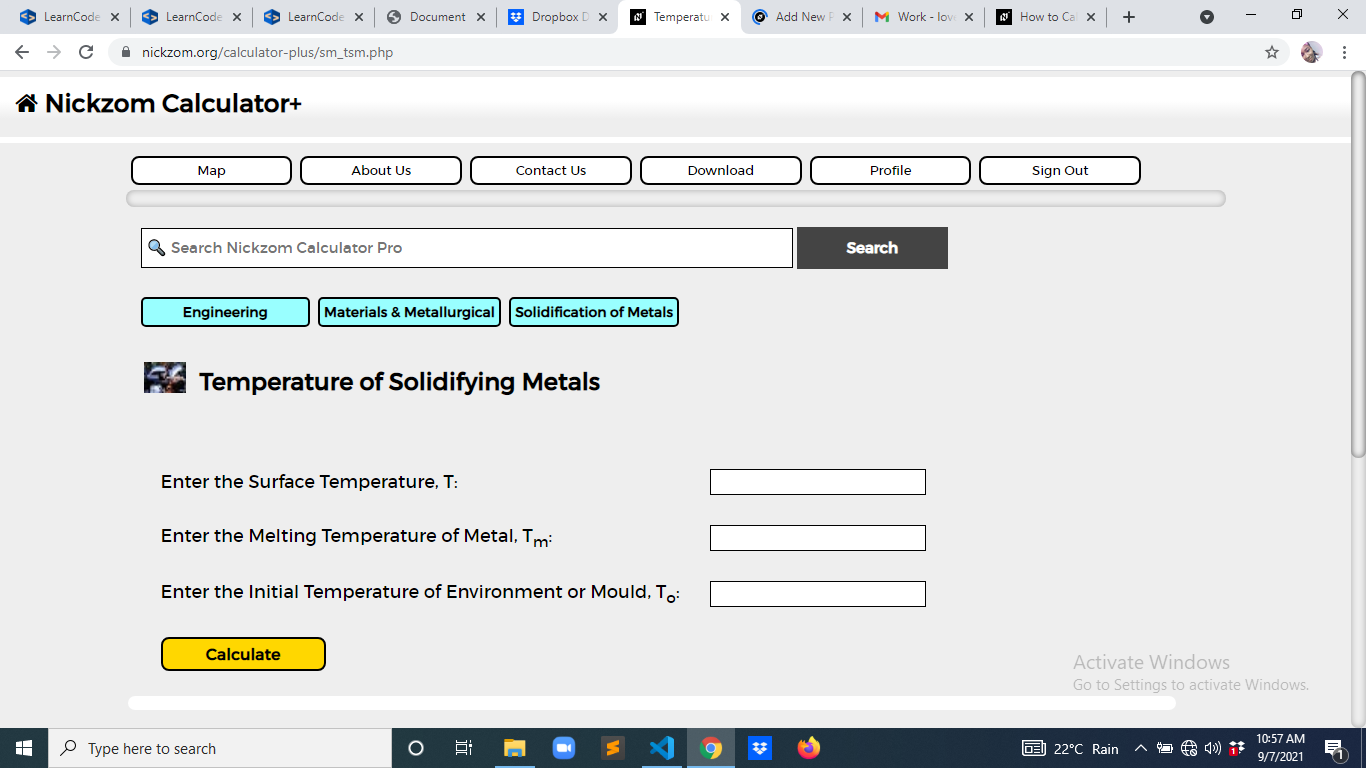Now, enter the values appropriately and accordingly for the parameters as required by the Surface Temperature (T) is 12, Melting Temperature of Metal (Tm) is 9 and Initial Temperature of Environment or Mould (To) is 10.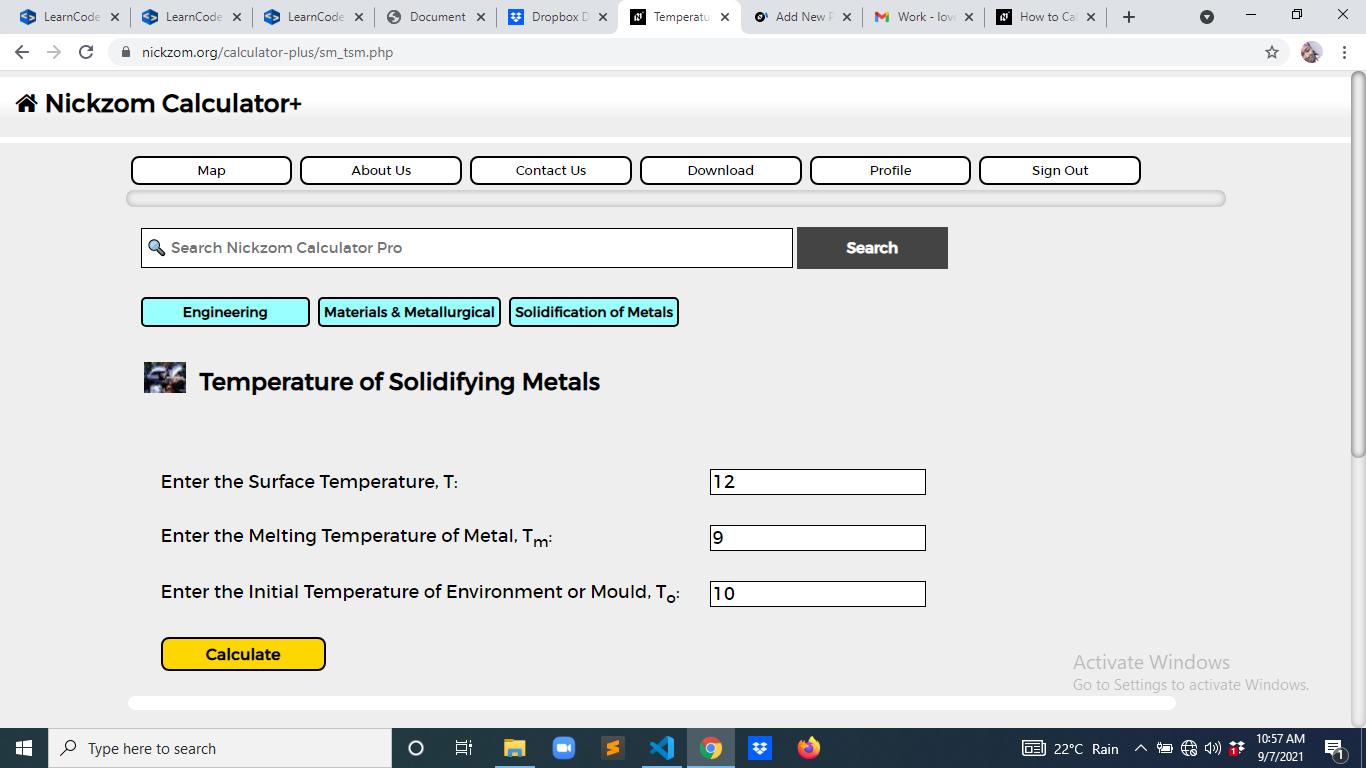Finally, Click on Calculate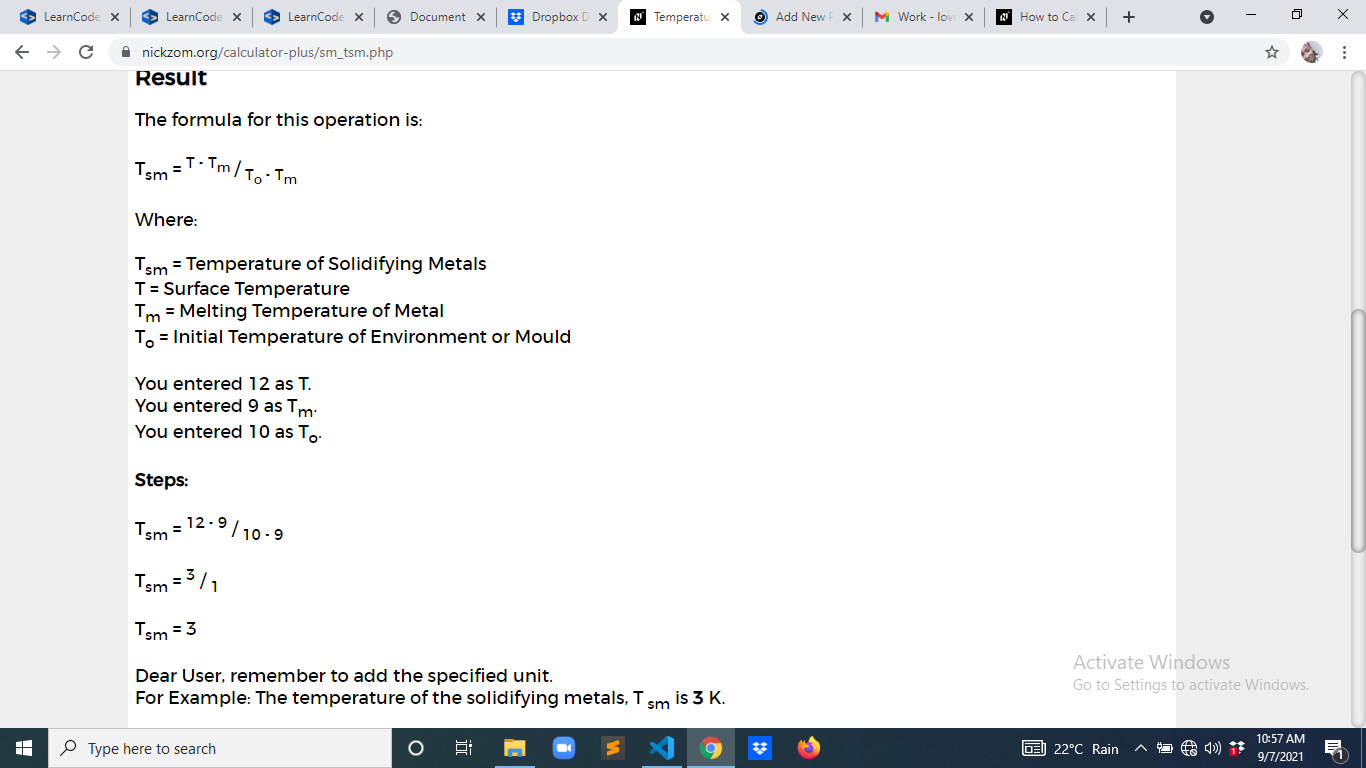As you can see from the screenshot above, Nickzom Calculator– The Calculator Encyclopedia solves for the temperature of solidifying metals and presents the formula, workings and steps too.